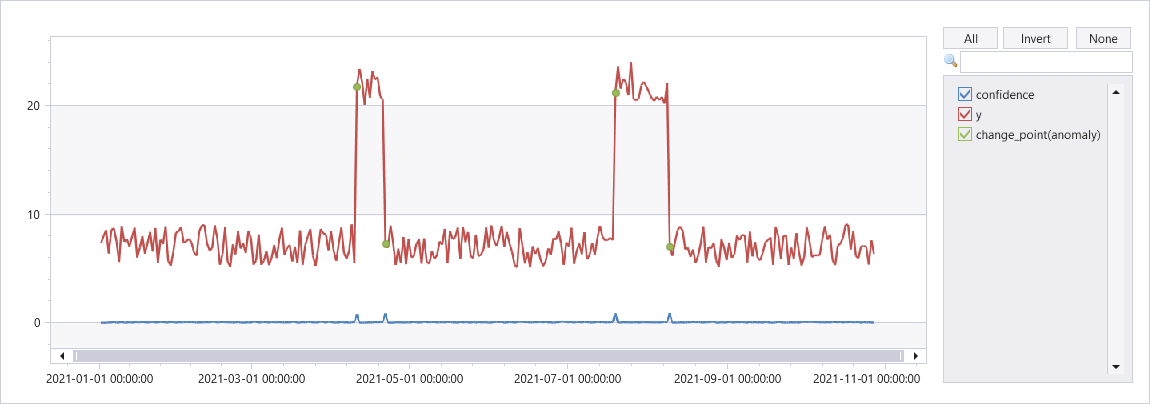# series_uv_change_points_fl()

The function `series_uv_change_points_fl()` is a user-defined function (UDF) that finds change points in time series by calling the Univariate Anomaly Detection API, part of Azure Cognitive Services. The function accepts a limited set of time series as numerical dynamic arrays, the change point detection threshold, and the minimum size of the stable trend window. Each time series is converted into the required JSON format and posts it to the Anomaly Detector service endpoint. The service response contains dynamic arrays of change points, their respective confidence, and the detected seasonality.

Note

Consider using the native function series_decompose_anomalies() which is more scalable and runs faster.

## Syntax

`T | invoke series_uv_change_points_fl(`y_series [`,` score_threshold [`,` trend_window [`,` tsid]]]`)`

## Parameters

Name Type Required Description
y_series string The name of the input table column containing the values of the series to be anomaly detected.
score_threshold real A value specifying the minimum confidence to declare a change point. Each point whose confidence is above the threshold is defined as a change point. Default value: 0.9
trend_window integer A value specifying the minimal window size for robust calculation of trend changes. Default value: 5
tsid string The name of the input table column containing the time series ID. Can be omitted when analyzing a single time series.

## Function definition

You can define the function by either embedding its code as a query-defined function, or creating it as a stored function in your database, as follows:

Define the function using the following let statement. No permissions are required. In the following function definition, replace `YOUR-AD-RESOURCE-NAME` in the uri and `YOUR-KEY` in the `Ocp-Apim-Subscription-Key` of the header with your Anomaly Detector resource name and key.

Important

A let statement can't run on its own. It must be followed by a tabular expression statement. To run a working example of `series_uv_change_points_fl()`, see Example.

``````let series_uv_change_points_fl=(tbl:(*), y_series:string, score_threshold:real=0.9, trend_window:int=5, tsid:string='_tsid')
{
let kwargs = bag_pack('y_series', y_series, 'score_threshold', score_threshold, 'trend_window', trend_window);
let code = ```if 1:
import json
y_series = kargs["y_series"]
score_threshold = kargs["score_threshold"]
trend_window = kargs["trend_window"]
json_str = []
for i in range(len(df)):
row = df.iloc[i, :]
ts = [{'value':row[y_series][j]} for j in range(len(row[y_series]))]
json_data = {'series': ts, "threshold":score_threshold, "stableTrendWindow": trend_window}     # auto-detect period, or we can force 'period': 84
json_str = json_str + [json.dumps(json_data)]
result = df
result['json_str'] = json_str
```;
tbl
| evaluate python(typeof(*, json_str:string), code, kwargs)
| extend _tsid = column_ifexists(tsid, 1)
| partition by _tsid (
project json_str
| project period=ResponseBody.period, change_point=series_add(0, ResponseBody.isChangePoint), confidence=ResponseBody.confidenceScores
| extend _tsid=toscalar(_tsid)
)
};
// Write your query to use the function here.
``````

## Example

The following example uses the invoke operator to run the function.

To use a query-defined function, invoke it after the embedded function definition.

``````let series_uv_change_points_fl=(tbl:(*), y_series:string, score_threshold:real=0.9, trend_window:int=5, tsid:string='_tsid')
{
let kwargs = bag_pack('y_series', y_series, 'score_threshold', score_threshold, 'trend_window', trend_window);
let code = ```if 1:
import json
y_series = kargs["y_series"]
score_threshold = kargs["score_threshold"]
trend_window = kargs["trend_window"]
json_str = []
for i in range(len(df)):
row = df.iloc[i, :]
ts = [{'value':row[y_series][j]} for j in range(len(row[y_series]))]
json_data = {'series': ts, "threshold":score_threshold, "stableTrendWindow": trend_window}     # auto-detect period, or we can force 'period': 84
json_str = json_str + [json.dumps(json_data)]
result = df
result['json_str'] = json_str
```;
tbl
| evaluate python(typeof(*, json_str:string), code, kwargs)
| extend _tsid = column_ifexists(tsid, 1)
| partition by _tsid (
project json_str
| project period=ResponseBody.period, change_point=series_add(0, ResponseBody.isChangePoint), confidence=ResponseBody.confidenceScores
| extend _tsid=toscalar(_tsid)
)
};
let ts = range x from 1 to 300 step 1
| extend y=iff(x between (100 .. 110) or x between (200 .. 220), 20, 5)
| extend ts=datetime(2021-01-01)+x*1d
| extend y=y+4*rand()
| summarize ts=make_list(ts), y=make_list(y)
| extend sid=1;
ts
| invoke series_uv_change_points_fl('y', 0.8, 10, 'sid')
| join ts on \$left._tsid == \$right.sid
| project-away _tsid
| project-reorder y, *      //  just to visualize the anomalies on top of y series
| render anomalychart with(xcolumn=ts, ycolumns=y, confidence, anomalycolumns=change_point)
``````

Output

The following graph shows change points on a time series.This feature isn't supported.